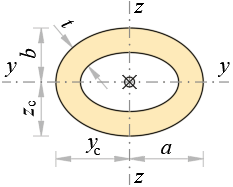### Geometrical Properties of Elliptical PipeDimensions

a = m, b = m

t = m

a1 = at m

b1 = bt m

Area

A = π·(a·ba1·b1) m2

Centroid

yc = a m, zc = b m

Perimeter (approx.)

P = π·(3·(a + b) –  (3·a + b)·(a + 3·b)) m

Second moments of area

Iy = π4·(a·b3a1·b13) m4

Iz = π4·(b·a3b1·a13) m4

Polar moment of area

Ix = Iy + Iz m4

Torsional constant

#if

k = b1b

#else

k = a1a

#end if

It = π·a3·b3a2 + b2·(1 – k4) m4

Torsional section modulus

#if

Wt = π·a·b22·(1 – k4) m3

#else

Wt = π·b·a22·(1 – k4) m3

#end if